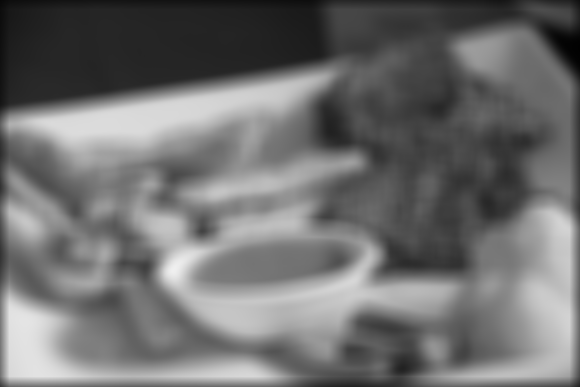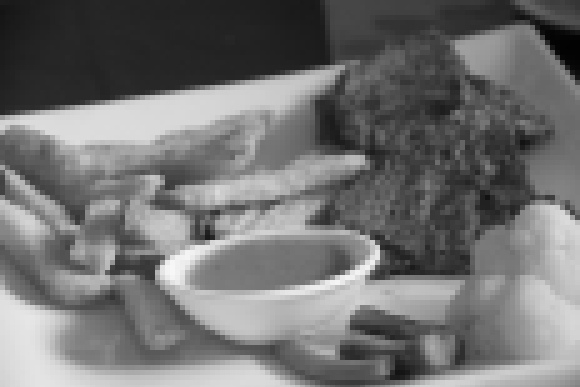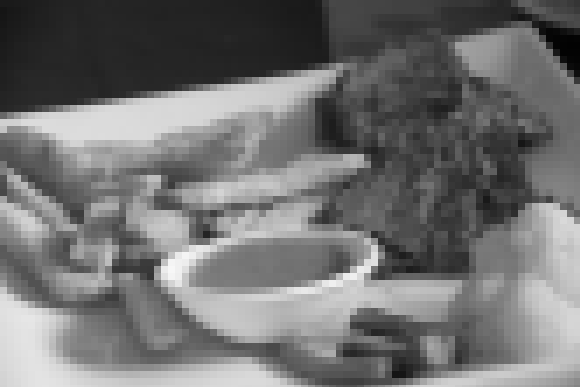# 【MATLAB】P图神器，初露锋芒：第一周作业(剧透)

Write a computer program capable of reducing the number of intensity levels in an image from 256 to 2, in integer powers of 2. The desired number of intensity levels needs to be a variable input to your program.
Using any programming language you feel comfortable with (it is though recommended to use the provided free Matlab), load an image and then perform a simple spatial 3x3 average of image pixels. In other words, replace the value of every pixel by the average of the values in its 3x3 neighborhood. If the pixel is located at (0,0), this means averaging the values of the pixels at the positions (-1,1), (0,1), (1,1), (-1,0), (0,0), (1,0), (-1,-1), (0,-1), and (1,-1). Be careful with pixels at the image boundaries. Repeat the process for a 10x10 neighborhood and again for a 20x20 neighborhood. Observe what happens to the image (we will discuss this in more details in the very near future, about week 3).
Rotate the image by 45 and 90 degrees (Matlab provides simple command lines for doing this).
For every 3×3 block of the image (without overlapping), replace all corresponding 9 pixels by their average. This operation simulates reducing the image spatial resolution. Repeat this for 5×5 blocks and 7×7 blocks. If you are using Matlab, investigate simple command lines to do this important operation.
=======================

## 实验用图=======================

## 用得着的Matlab说明文档

blockproc
http://www.mathworks.cn/cn/help/images/ref/blockproc.html
Performing Distinct Block Operations
http://www.mathworks.cn/cn/help/images/performing-distinct-block-operations.html
Performing Sliding Neighborhood Operations
http://www.mathworks.cn/cn/help/images/performing-sliding-neighborhood-operations.html
nlfilter
http://www.mathworks.cn/cn/help/images/ref/nlfilter.html
Local Functions
http://www.mathworks.cn/cn/help/matlab/matlab_prog/local-functions.html
idivide
http://www.mathworks.cn/cn/help/matlab/ref/idivide.html
Loop Control Statements
http://www.mathworks.cn/cn/help/matlab/matlab_prog/loop-control-statements.html
Matrix Indexing
http://www.mathworks.cn/cn/help/matlab/math/matrix-indexing.html
mean
http://www.mathworks.cn/cn/help/matlab/ref/mean.html
mean2
http://www.mathworks.cn/cn/help/images/ref/mean2.html
sum
http://www.mathworks.cn/cn/help/matlab/ref/sum.html
imrotate
http://www.mathworks.cn/cn/help/images/ref/imrotate.html

=======================

## 读取图片，转成灰度图

```origin_im = imread('F:/dip/goof.jpg'); %读取图片,要修改图片路径为自己的图片才能执行哦
gray_im = rgb2gray(origin_im); %转成灰度图
```Week1所需要的处理，在灰度图中比较好操作，所以先把图片转换成灰度。(马上没有食欲了...)
=======================

## 逐次改变intensity

```
image_size = size(gray_im);
out_gif = uint8( zeros( image_size(1),image_size(2),1,8));
for n = 1:8
intensity = 2^n;
intensity_reduced = ( gray_im / ( 256/(intensity-1) ) ) * ( 256/(intensity-1) );
out_gif(:,:,1,9-n) = intensity_reduced;
end
imwrite(out_gif,'F:\dip\goof_intensity.gif','LoopCount',Inf);

```Intensity逐渐减少，就是这个效果的~
=======================

## 平滑处理(Overlap average)

=======================

```
fun = @(x) uint8(mean2(x(:)));
overlap_avg_3 = nlfilter(gray_im,[3 3],fun);
overlap_avg_10 = nlfilter(gray_im,[10 10],fun);
overlap_avg_20 = nlfilter(gray_im,[20 20],fun);
figure,imshow(gray_im),figure,imshow(overlap_avg_3),figure,imshow(overlap_avg_10),figure,imshow(overlap_avg_20);

```=======================

## 图像旋转(Rotation)

```
r45 = imrotate(gray_im,45);
r90 = imrotate(gray_im,90);
figure,imshow(r45),figure,imshow(r90);

```=======================

## Non-overlap average

=======================

```
fun = @(block_struct) uint8( mean2(block_struct.data(:))  * ones(block_struct.blockSize) );
block_avg_3 = blockproc(gray_im,[3 3],fun);
block_avg_5 = blockproc(gray_im,[5 5],fun);
block_avg_7 = blockproc(gray_im,[7 7],fun);
figure,imshow(gray_im),figure,imshow(block_avg_3),figure,imshow(block_avg_5),figure,imshow(block_avg_7);

```